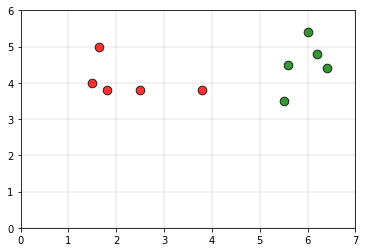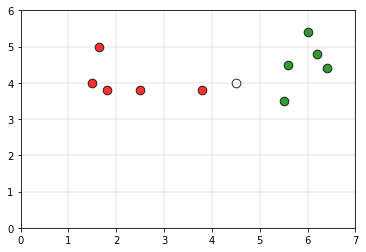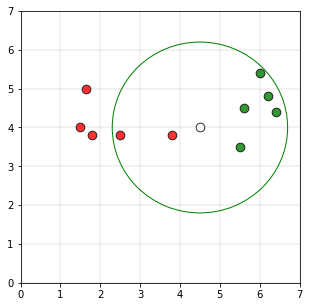# r-Nearest neighbors

r-Nearest neighbors is a modified version of the k-nearest neighbors. The issue with k-nearest neighbors is the choice of k. A smaller k, the classifier would be more sensitive to outliers. If the value of k is large, then the classifier would be including many points from other classes. It is from this logic that we get the r near neighbors algorithm.

Intuition:
Consider the following data, as the training set.The green color points belong to class 0 and the red color points belong to class 1.
Consider the white point P as the query point whoseIf we take the radius of the circle is 2.2 units and if a circle is drawn using the point P as the center of the circle, the plot would be as followsAs the number of points in the circle belonging to class 1 (5 points) are greater than the number of points belonging to class 0 (2 points)

Algorithm:

Step 1: Given the point P, determine the sub-set of data that lies in the ball of radius r centered at P,

Br (P) = { Xi ∊ X | dist( P, Xi ) ≤ r }

Step 2: If Br (P) is empty, then output the majority class of the entire data set.

Step 3: If Br (P) is not empty, output the majority class of the data points in it.

Implementation of the r radius neighbors algorithm is as follows::

## C/C++

 `// C++ program to implement the ` `// r nearest neighbours algorithm. ` `#include ` `using` `namespace` `std; ` ` `  `struct` `Point ` `{ ` `    ``// Class of point ` `    ``int` `val;  ` `     `  `    ``// Co-ordinate of point ` `    ``double` `x, y;  ` `}; ` ` `  `// This function classifies the point p using ` `// r k neareast neighbour algorithm. It assumes only ` `// two groups and returns 0 if p belongs to class 0, else ` `// 1 (belongs to class 1). ` `int` `rNN(Point arr[], ``int` `n, ``float` `r, Point p) ` `{ ` `    ``// frequency of group 0 ` `    ``int` `freq1 = 0;  ` `    ``// frequency of group 1 ` `    ``int` `freq2 = 0;  ` ` `  `    ``// Check if the distance is less than r ` `    ``for` `(``int` `i = 0; i < n; i++) ` `    ``{ ` ` `  `        ``if` `((``sqrt``((arr[i].x - p.x) * (arr[i].x - p.x) +  ` `        ``(arr[i].y - p.y) * (arr[i].y - p.y))) <= r) ` `        ``{ ` `            ``if` `(arr[i].val == 0) ` `                ``freq1++; ` `            ``else` `if` `(arr[i].val == 1) ` `                ``freq2++; ` `        ``} ` `    ``} ` `    ``return` `(freq1 > freq2 ? 0 : 1); ` `} ` ` `  `// Driver code ` `int` `main() ` `{ ` `    ``// Number of data points ` `    ``int` `n = 10;  ` `    ``Point arr[n]; ` ` `  `    ``arr.x = 1.5; ` `    ``arr.y = 4; ` `    ``arr.val = 0; ` ` `  `    ``arr.x = 1.8; ` `    ``arr.y = 3.8; ` `    ``arr.val = 0; ` ` `  `    ``arr.x = 1.65; ` `    ``arr.y = 5; ` `    ``arr.val = 0; ` ` `  `    ``arr.x = 2.5; ` `    ``arr.y = 3.8; ` `    ``arr.val = 0; ` ` `  `    ``arr.x = 3.8; ` `    ``arr.y = 3.8; ` `    ``arr.val = 0; ` ` `  `    ``arr.x = 5.5; ` `    ``arr.y = 3.5; ` `    ``arr.val = 1; ` ` `  `    ``arr.x = 5.6; ` `    ``arr.y = 4.5; ` `    ``arr.val = 1; ` ` `  `    ``arr.x = 6; ` `    ``arr.y = 5.4; ` `    ``arr.val = 1; ` ` `  `    ``arr.x = 6.2; ` `    ``arr.y = 4.8; ` `    ``arr.val = 1; ` ` `  `    ``arr.x = 6.4; ` `    ``arr.y = 4.4; ` `    ``arr.val = 1; ` ` `  `    ``// Query point ` `    ``Point p; ` `    ``p.x = 4.5; ` `    ``p.y = 4; ` ` `  `    ``// Parameter to decide the class of the query point ` `    ``float` `r = 2.2; ` `    ``printf``(``"The value classified to query point"` `           ``" is: %d.\n"``, rNN(arr, n, r, p)); ` `    ``return` `0; ` `} `

## Python3

 `# Python3 program to implement the  ` `# r nearest neighbours algorithm.  ` `import` `math  ` ` `  `def` `rNN(points, p, r ``=` `2.2``):  ` `        ``'''  ` `        ``This function classifies the point p using  ` `        ``r k neareast neighbour algorithm. It assumes only   ` `        ``two groups and returns 0 if p belongs to class 0, else  ` `        ``1 (belongs to class 1).  ` ` `  `        ``Parameters -  ` `                ``points : Dictionary of training points having two ` `                         ``keys - 0 and 1. Each class have a list of ` `                         ``training data points belonging to them  ` ` `  `                ``p : A tuple, test data point of form (x, y)  ` `                ``k : radius of the r nearest neighbors  ` `        ``'''` ` `  `        ``freq1 ``=` `0` `        ``freq2 ``=` `0` `        ``for` `group ``in` `points:  ` `                ``for` `feature ``in` `points[group]:  ` `                        ``if` `math.sqrt((feature[``0``]``-``p[``0``])``*``*``2` `+`  `                                     ``(feature[``1``]``-``p[``1``])``*``*``2``) <``=` `r: ` `                                ``if` `group ``=``=` `0``: ` `                                        ``freq1 ``+``=` `1`                       `                                ``elif` `group ``=``=` `1``:  ` `                                        ``freq2 ``+``=` `1`  `                         `  `        ``return` `0` `if` `freq1>freq2 ``else` `1` ` `  `# Driver function  ` `def` `main():  ` ` `  `        ``# Dictionary of training points having two keys - 0 and 1  ` `        ``# key 0 have points belong to class 0  ` `        ``# key 1 have points belong to class 1  ` ` `  `        ``points ``=` `{``0``:[(``1.5``, ``4``), (``1.8``, ``3.8``), (``1.65``, ``5``), (``2.5``, ``3.8``), (``3.8``, ``3.8``)],  ` `                  ``1``:[(``5.5``, ``3.5``), (``5.6``, ``4.5``), (``6``, ``5.4``), (``6.2``, ``4.8``), (``6.4``, ``4.4``)]}  ` ` `  `        ``# query point p(x, y)  ` `        ``p ``=` `(``4.5``, ``4``)  ` ` `  `        ``# Parameter to decide the class of the query point  ` `        ``r ``=` `2.2` ` `  `        ``print``(``"The value classified to query point is: {}"``.``format``( ` `                ``rNN(points, p, r)))  ` ` `  `if` `__name__ ``=``=` `'__main__'``:  ` `        ``main()  `

Output:

```The value classified to query point is: 1.
```

Other techniques like kd-tree, locality sensitive hashing can be used to reduce the time complexity of finding the neighbors.

Applications:
This algorithm can be used to identify outliers. If a pattern does not have any similarity with the patterns within the radius chosen, it can be identified as an outlier.

Attention geek! Strengthen your foundations with the Python Programming Foundation Course and learn the basics.

To begin with, your interview preparations Enhance your Data Structures concepts with the Python DS Course.

My Personal Notes arrow_drop_upCheck out this Author's contributed articles.

If you like GeeksforGeeks and would like to contribute, you can also write an article using contribute.geeksforgeeks.org or mail your article to contribute@geeksforgeeks.org. See your article appearing on the GeeksforGeeks main page and help other Geeks.

Please Improve this article if you find anything incorrect by clicking on the "Improve Article" button below.